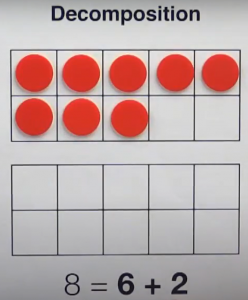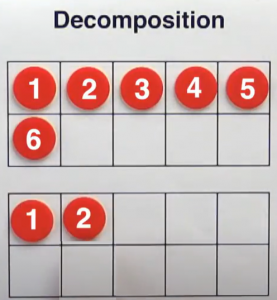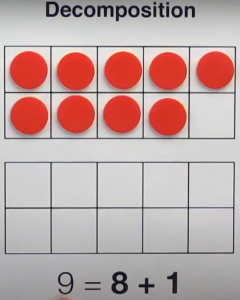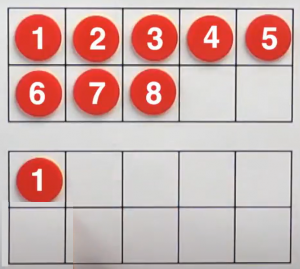# Lesson 12 Break numbers apart or decomposition (Worksheet)

Decomposition is just a fancy word for breaking numbers apart and it is easy to do. Let’s take a look. In the decomposition example, we start with eight counters and we are asked to break it apart into six plus two.How do we do that? We take the eight counters move the last two counters down and now we have six plus two.Here is another example.Take nine and decompose it into eight plus one. So take one counter and now we have eight plus one.Let’s learn how to do that on paper. Here we are going to take eight and decompose it into six plus two. Here are the original eight counters to decompose it we cross off the last two and move them down into the bottom ten frame and now we have six plus two. Eight equals six plus two. Let’s take a look at three more examples.

## Worksheets for the lessonМатематичні настільні ігри